# RD Sharma Solutions for Class 11 Chapter 23 - The Straight Lines Exercise 23.10

Here, we shall learn the point of intersection of two lines, with suitable examples, for students to understand the concepts clearly. The solutions created are considered to be very useful when you are preparing for the CBSE Class 11 Maths exams. Here, we bring to you detailed solutions to the exercises of RD Sharma Class 11 Maths Solutions of Chapter 23. Subject matter experts who created the solutions have compiled these questions for students to revise from the Chapter 23 of RD Sharma Textbook. Students can easily download the solutions pdf from the links given below and start practising offline.

## Download the pdf of RD Sharma Solutions for Class 11 Maths Exercise 23.10 Chapter 23 – The Straight Lines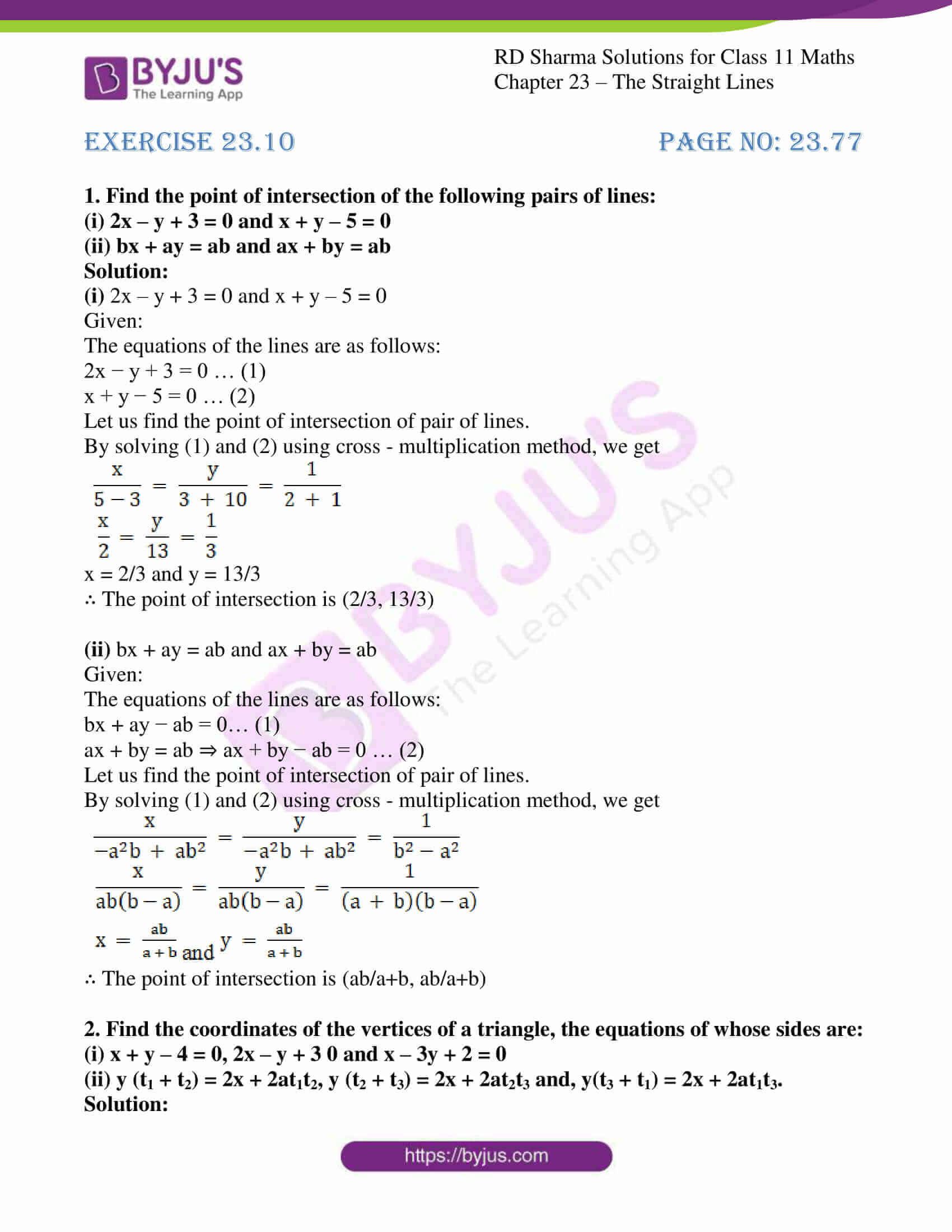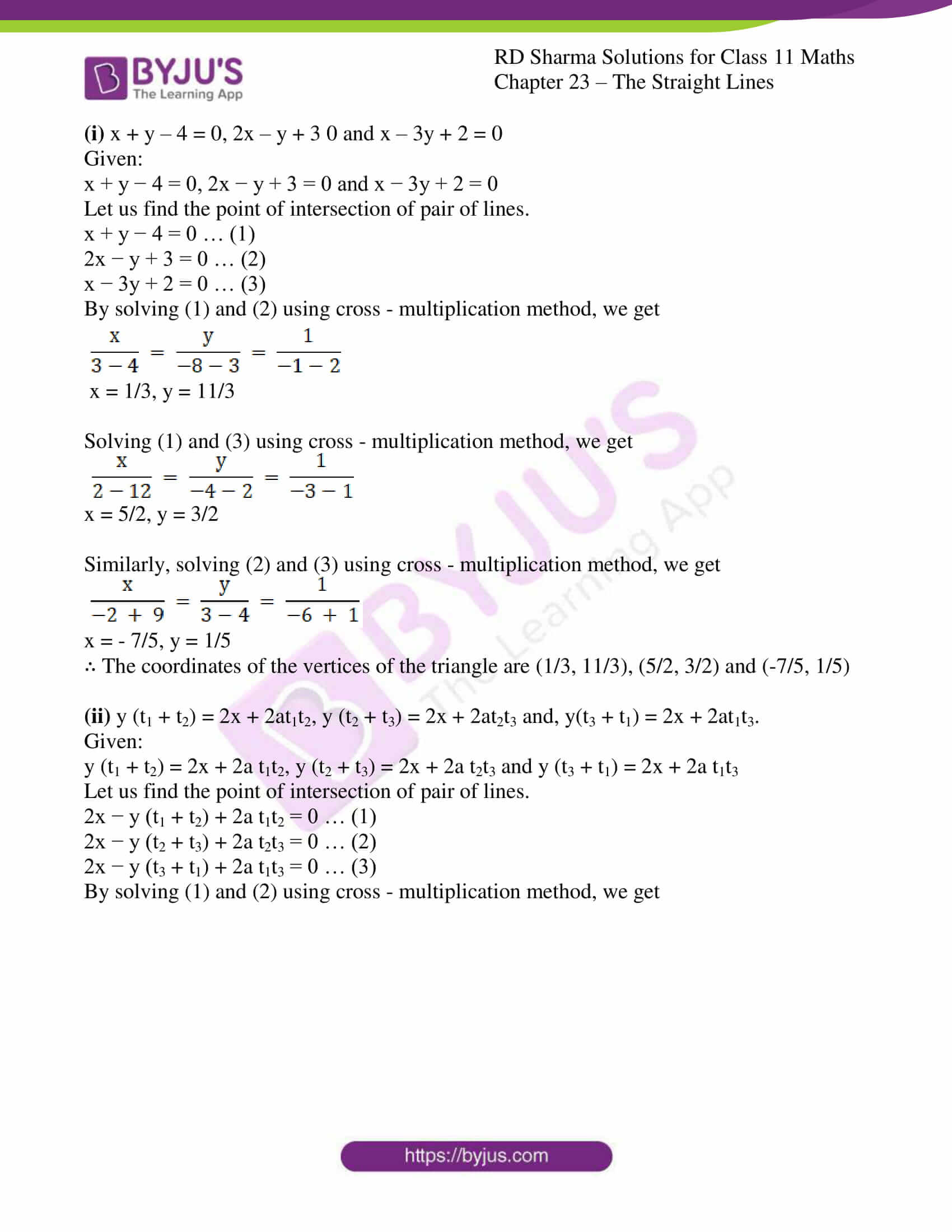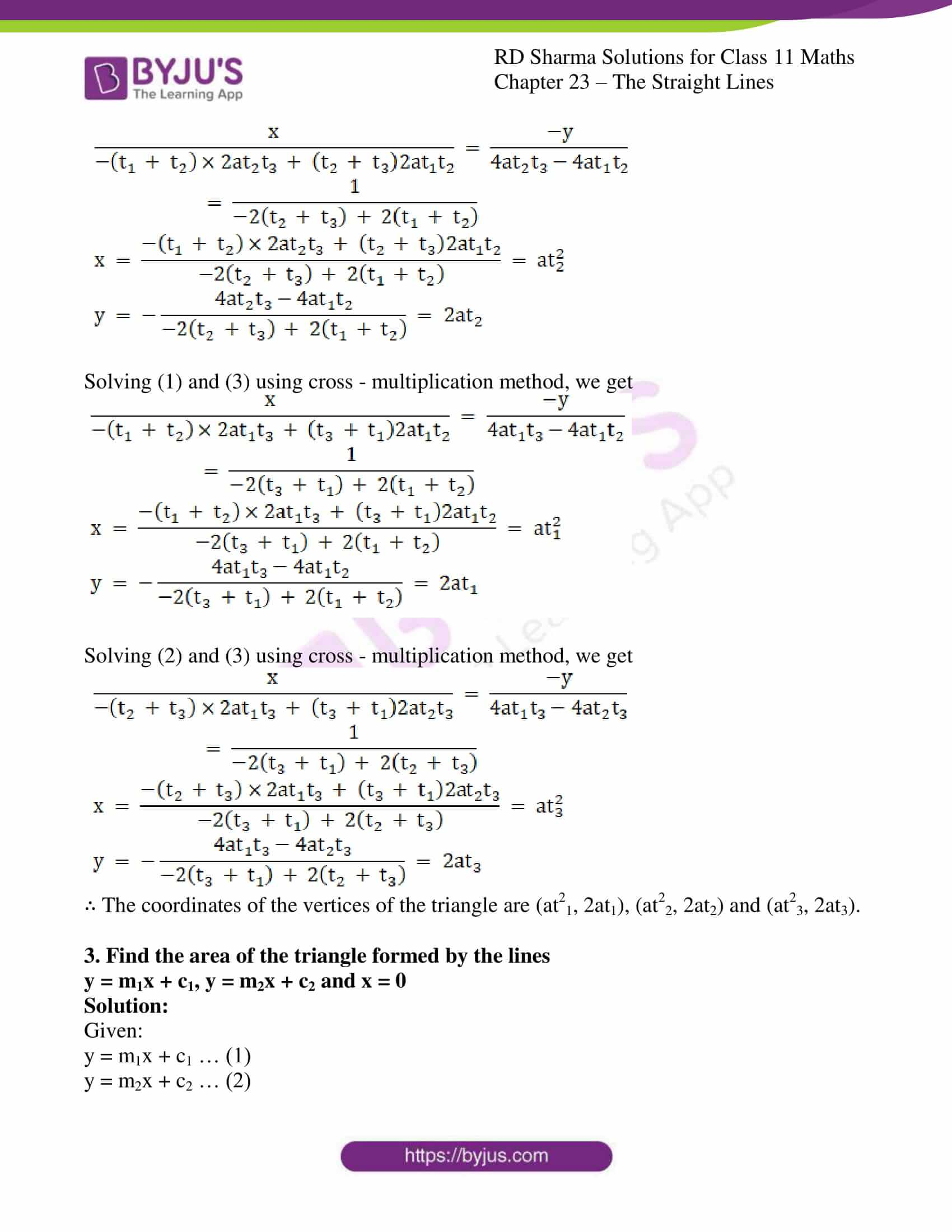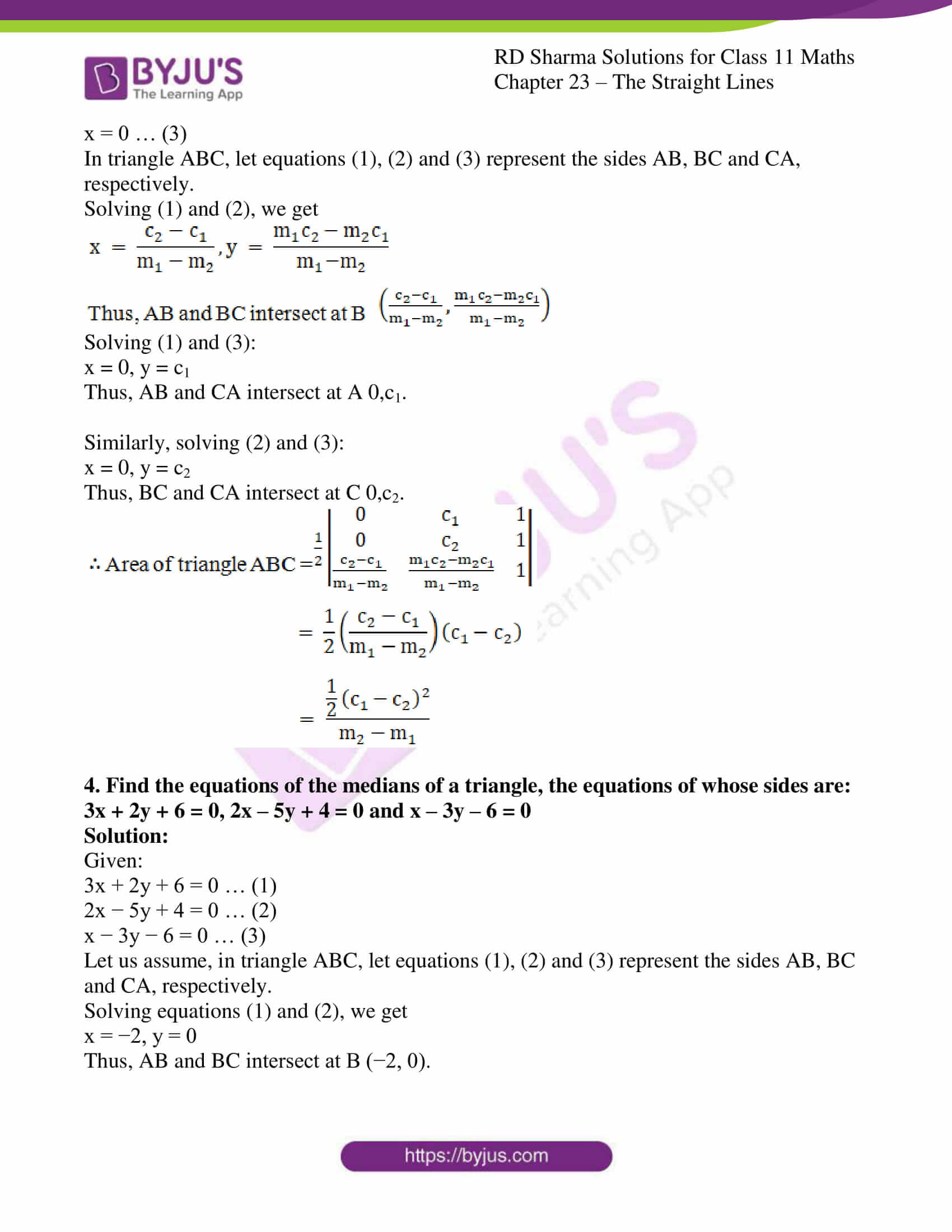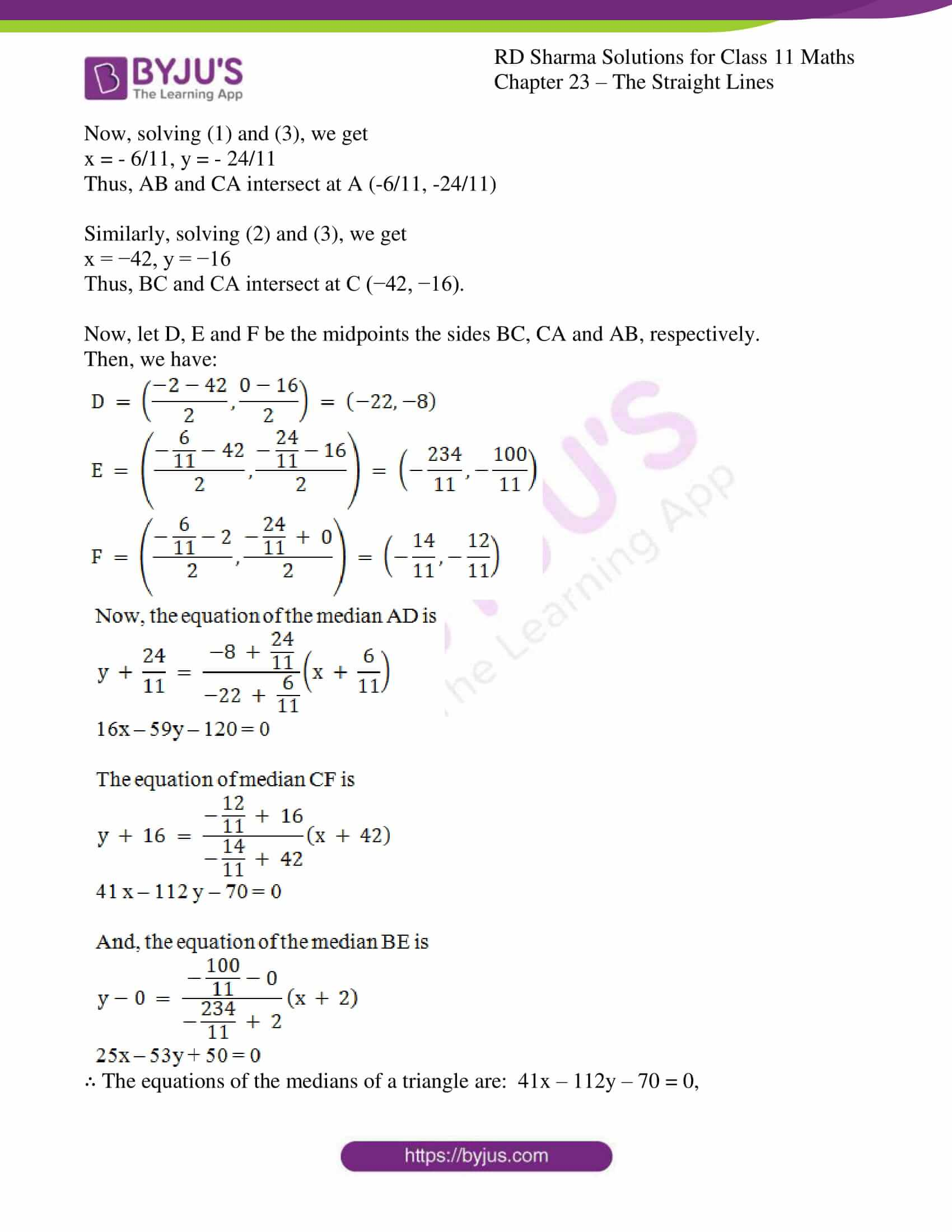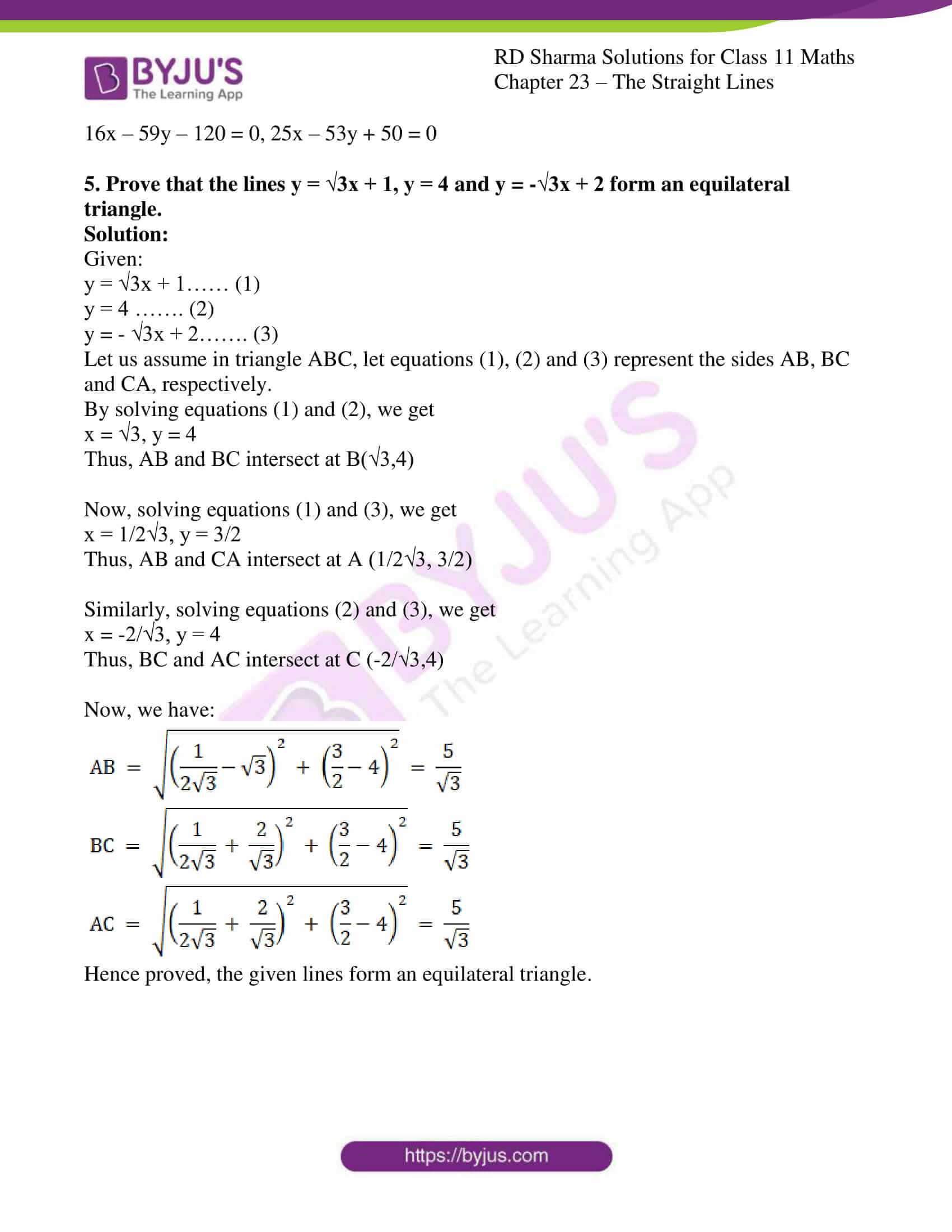### Also, access other exercises of RD Sharma Solutions for Class 11 Maths Chapter 23 – The Straight Lines

Exercise 23.1 Solutions

Exercise 23.2 Solutions

Exercise 23.3 Solutions

Exercise 23.4 Solutions

Exercise 23.5 Solutions

Exercise 23.6 Solutions

Exercise 23.7 Solutions

Exercise 23.8 Solutions

Exercise 23.9 Solutions

Exercise 23.11 Solutions

Exercise 23.12 Solutions

Exercise 23.13 Solutions

Exercise 23.14 Solutions

Exercise 23.15 Solutions

Exercise 23.16 Solutions

Exercise 23.17 Solutions

Exercise 23.18 Solutions

Exercise 23.19 Solutions

### Access answers to RD Sharma Solutions for Class 11 Maths Exercise 23.10 Chapter 23 – The Straight Lines

#### EXERCISE 23.10 PAGE NO: 23.77

1. Find the point of intersection of the following pairs of lines:
(i) 2x – y + 3 = 0 and x + y – 5 = 0

(ii) bx + ay = ab and ax + by = ab

Solution:

(i) 2x – y + 3 = 0 and x + y – 5 = 0

Given:

The equations of the lines are as follows:

2x − y + 3 = 0 … (1)

x + y − 5 = 0 … (2)

Let us find the point of intersection of pair of lines.

By solving (1) and (2) using cross – multiplication method, we get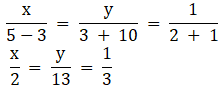x = 2/3 and y = 13/3

∴ The point of intersection is (2/3, 13/3)

(ii) bx + ay = ab and ax + by = ab

Given:

The equations of the lines are as follows:

bx + ay − ab = 0… (1)

ax + by = ab ⇒ ax + by − ab = 0 … (2)

Let us find the point of intersection of pair of lines.

By solving (1) and (2) using cross – multiplication method, we get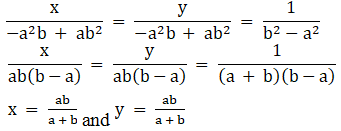∴ The point of intersection is (ab/a+b, ab/a+b)

2. Find the coordinates of the vertices of a triangle, the equations of whose sides are: (i) x + y – 4 = 0, 2x – y + 3 0 and x – 3y + 2 = 0

(ii) y (t1 + t2) = 2x + 2at1t2, y (t2 + t3) = 2x + 2at2t3 and, y(t3 + t1) = 2x + 2at1t3.

Solution:

(i) x + y – 4 = 0, 2x – y + 3 0 and x – 3y + 2 = 0

Given:

x + y − 4 = 0, 2x − y + 3 = 0 and x − 3y + 2 = 0

Let us find the point of intersection of pair of lines.

x + y − 4 = 0 … (1)

2x − y + 3 = 0 … (2)

x − 3y + 2 = 0 … (3)

By solving (1) and (2) using cross – multiplication method, we get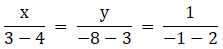x = 1/3, y = 11/3

Solving (1) and (3) using cross – multiplication method, we get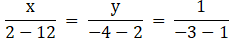x = 5/2, y = 3/2

Similarly, solving (2) and (3) using cross – multiplication method, we get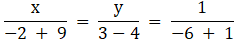x = – 7/5, y = 1/5

∴ The coordinates of the vertices of the triangle are (1/3, 11/3), (5/2, 3/2) and (-7/5, 1/5)

(ii) y (t1 + t2) = 2x + 2at1t2, y (t2 + t3) = 2x + 2at2t3 and, y(t3 + t1) = 2x + 2at1t3.

Given:

y (t1 + t2) = 2x + 2a t1t2, y (t2 + t3) = 2x + 2a t2t3 and y (t3 + t1) = 2x + 2a t1t3

Let us find the point of intersection of pair of lines.

2x − y (t1 + t2) + 2a t1t2 = 0 … (1)

2x − y (t2 + t3) + 2a t2t3 = 0 … (2)

2x − y (t3 + t1) + 2a t1t3 = 0 … (3)

By solving (1) and (2) using cross – multiplication method, we get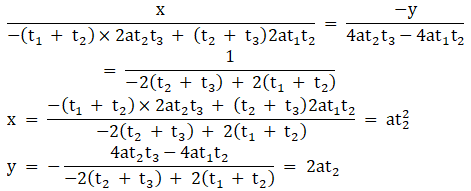Solving (1) and (3) using cross – multiplication method, we get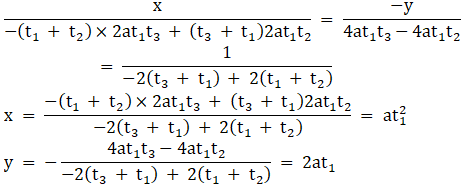Solving (2) and (3) using cross – multiplication method, we get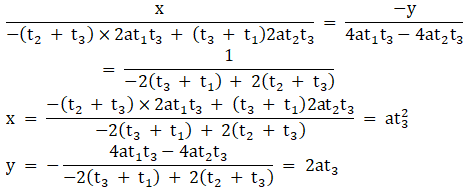∴ The coordinates of the vertices of the triangle are (at21, 2at1), (at22, 2at2) and (at23, 2at3).

3. Find the area of the triangle formed by the lines
y = m1x + c1, y = m2x + c2 and x = 0

Solution:

Given:

y = m1x + c1 … (1)

y = m2x + c2 … (2)

x = 0 … (3)

In triangle ABC, let equations (1), (2) and (3) represent the sides AB, BC and CA, respectively.

Solving (1) and (2), we get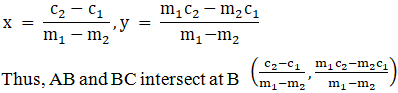Solving (1) and (3):

x = 0, y = c1

Thus, AB and CA intersect at A 0,c1.

Similarly, solving (2) and (3):

x = 0, y = c2

Thus, BC and CA intersect at C 0,c2.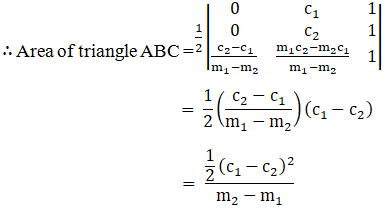4. Find the equations of the medians of a triangle, the equations of whose sides are:
3x + 2y + 6 = 0, 2x – 5y + 4 = 0 and x – 3y – 6 = 0

Solution:

Given:

3x + 2y + 6 = 0 … (1)

2x − 5y + 4 = 0 … (2)

x − 3y − 6 = 0 … (3)

Let us assume, in triangle ABC, let equations (1), (2) and (3) represent the sides AB, BC and CA, respectively.

Solving equations (1) and (2), we get

x = −2, y = 0

Thus, AB and BC intersect at B (−2, 0).

Now, solving (1) and (3), we get

x = – 6/11, y = – 24/11

Thus, AB and CA intersect at A (-6/11, -24/11)

Similarly, solving (2) and (3), we get

x = −42, y = −16

Thus, BC and CA intersect at C (−42, −16).

Now, let D, E and F be the midpoints the sides BC, CA and AB, respectively.

Then, we have: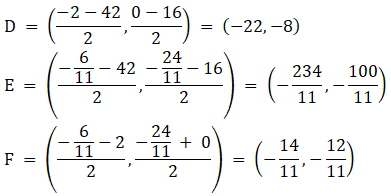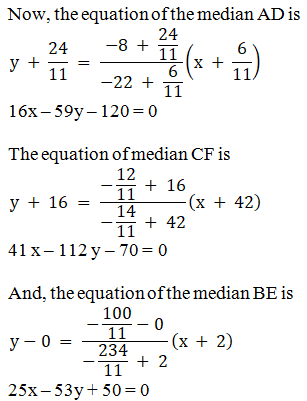∴ The equations of the medians of a triangle are: 41x – 112y – 70 = 0,

16x – 59y – 120 = 0, 25x – 53y + 50 = 0

5. Prove that the lines y = √3x + 1, y = 4 and y = -√3x + 2 form an equilateral triangle.

Solution:

Given:

y = √3x + 1…… (1)

y = 4 ……. (2)

y = – √3x + 2……. (3)

Let us assume in triangle ABC, let equations (1), (2) and (3) represent the sides AB, BC and CA, respectively.

By solving equations (1) and (2), we get

x = √3, y = 4

Thus, AB and BC intersect at B(√3,4)

Now, solving equations (1) and (3), we get

x = 1/2√3, y = 3/2

Thus, AB and CA intersect at A (1/2√3, 3/2)

Similarly, solving equations (2) and (3), we get

x = -2/√3, y = 4

Thus, BC and AC intersect at C (-2/√3,4)

Now, we have: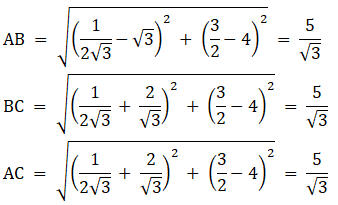Hence proved, the given lines form an equilateral triangle.